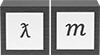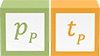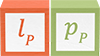# Symmetry

Natural symmetries feature prominently in New Foundation Model equations. Each formula conserves natural units of length, mass, and time, while showing how particles are distributed in space and time. These formulas demonstrate how mass, momentum, and energy are conserved.

The conservation of Planck energy is exemplified by the kinetic energy formulas for individual, elementary particles. A particle’s spatial distribution and temporal displacement may be considered dilutions of the conserved Planck energy over space and time, as described by the 2-part energy mechanism. The conservation of Planck energy is shown in the following formulas which consolidate both parts of the energy mechanism into a single time dimension–the particle’s oscillation period. Particles with rest mass have a cycle of two oscillation periods.

### rest mass

New Foundation Model operators show correlations across the unit dimensions in their description of elementary particles and systems, including field potentials. For example, the wavelength operator gives quantities of wavelength, inertial mass, and momentum that all have the same proportion to the Planck scale.

The following illustrations summarize the correlations across unit dimensions

## Length-mass symmetry

Length-mass symmetry is the conservation of length-mass across the two unit dimensions. Since length potential is the inverse of length units (so that Planck length is the maximum potential), the length-mass symmetry is represented by a single vertical line across both unit dimensions, making length and mass potentials equal.

Length-mass symmetry conserves 𝑙𝑃𝑚𝑃–the quantity 3.52 x 10-43 kgm–and produces an invariant quantity of wavelengthinertial mass in all particles whether or not they have rest mass.

ƛ𝑚 = 𝑙𝑃𝑚𝑃

### Potential### Invariant3.52 x 10-43 kgm

## Mass-momentum symmetry

Mass-momentum symmetry is an alternative expression of length-mass symmetry, but in traditional units of momentum. This symmetry incorporates a superfluous time dimension.

Mass-momentum symmetry conserves 𝑙𝑃𝑚𝑃 and 𝑝𝑃𝑡𝑃–the quantity 3.52 x 10-43 kgm–and produces invariant quantities of wavelengthinertial mass and momentumtime.

ƛ𝑚 = 𝑝𝑇𝜆

### Potential### Invariant3.52 x 10-43 kgm

3.52 x 10-43 kgm

## Momentum-energy symmetry

Momentum-energy symmetry describes both parts of the 2-part energy mechanism: wavelength and velocity. The symmetry results in equivalent 𝛽𝑝 and 𝛽𝑣 operators described by the New Foundation Model and generally notated as 𝑣2/𝑐2.

Momentum-energy symmetry conserves 𝑙𝑃𝑝𝑃 and 𝑡𝑃 𝐸𝑃, both of which are quantified by the reduced Planck constant–1.05 x 10-34 kgm2s-1. The symmetry produces invariant quantities of wavelengthmomentum and timeenergy.

ƛ𝑝 = 𝑇𝐸𝑘

### Potential### Invariant### Conserved quantity

1.05 x 10-34 kgm2s-1

1.05 x 10-34 kgm2s-1

The conservation of momentum-energy in particles with rest mass generates a current of.

Symmetries show that there is integrity in the physical construct of the universe. Correlations across unit dimensions are not abstract curiosities; they reflect the constraints of a material universe that behaves consistently in all places and at all times.

Symmetry plays an essential role in predicting the evolution of physical systems. The laws producing correlations of length, mass, and time constrain the permitted values of each unit dimension. A change in one dimension produces predictable quantities of the other two dimensions when the laws are known.